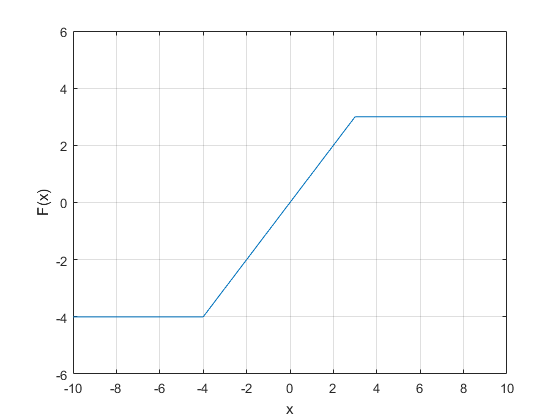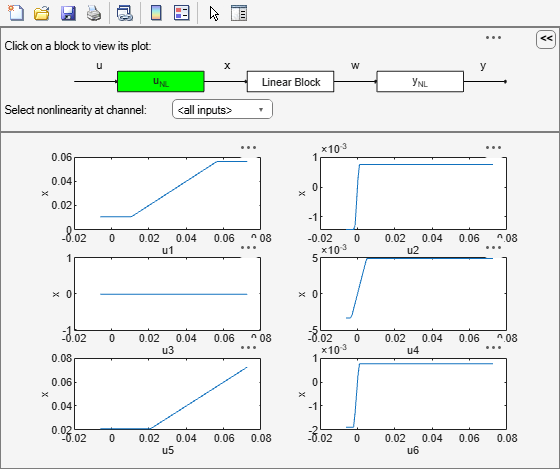# idSaturation

Create a saturation nonlinearity estimator object

## Syntax

```NL = idSaturation NL = idSaturation('LinearInterval',[a,b]) ```

## Description

`NL = idSaturation` creates a default saturation nonlinearity estimator object for estimating Hammerstein-Wiener models. The linear interval is set to `[NaN NaN]`. The initial value of the linear interval is determined from the estimation data range during estimation using `nlhw`. Use dot notation to customize the object properties, if needed.

`NL = idSaturation('LinearInterval',[a,b])` creates a saturation nonlinearity estimator object initialized with linear interval, `[a,b]`.

Alternatively, use `NL = idSaturation([a,b])`.

## Object Description

`idSaturation` is an object that stores the saturation nonlinearity estimator for estimating Hammerstein-Wiener models.

Use `idSaturation` to define a nonlinear function $y=F\left(x,\theta \right)$, where y and x are scalars, and θ represents the parameters a and b that define the linear interval, `[a,b]`.

The saturation nonlinearity function has the following characteristics:

For example, in the following plot, the linear interval is `[-4,3]`.The value `F(x)` is computed by `evaluate(NL,x)`, where `NL` is the `idSaturation` object.

For `idSaturation` object properties, see Properties.

## Examples

collapse all

`NL = idSaturation;`

Specify the linear interval.

`NL.LinearInterval = [-4,5];`

```load twotankdata; z = iddata(y,u,0.2,'Name','Two tank system'); z1 = z(1:1000);```

Create a saturation object with lower limit of 0 and upper limit of 5.

`InputNL = idSaturation('LinearInterval',[0 5]);`

Estimate model with no output nonlinearity.

`m = nlhw(z1,[2 3 0],InputNL,[]);`

`load motorizedcamera;`

Create an `iddata` object.

`z = iddata(y,u,0.02,'Name','Motorized Camera','TimeUnit','s');`

`z` is an `iddata` object with 6 inputs and 2 outputs.

Specify the model orders and delays.

`Orders = [ones(2,6),ones(2,6),ones(2,6)];`

Specify the same nonlinearity estimator for each input channel.

`InputNL = idSaturation;`

Specify different nonlinearity estimators for each output channel.

` OutputNL = [idDeadZone,idWaveletNetwork];`

Estimate the Hammerstein-Wiener model.

`sys = nlhw(z,Orders,InputNL,OutputNL);`

To see the shape of the estimated input and output nonlinearities, plot the nonlinearities.

`plot(sys)`Click on the input and output nonlinearity blocks on the top of the plot to see the nonlinearities.

## Input Arguments

collapse all

Linear interval of the saturation, specified as a 2–element row vector of doubles.

The saturation nonlinearity is initialized at the interval `[a,b]`. The interval values are adjusted to the estimation data by `nlhw`. To remove the lower limit, set `a` to `-Inf`. The lower limit is not adjusted during estimation. To remove the upper limit, set `b` to `Inf`. The upper limit is not adjusted during estimation.

When the interval is `[NaN NaN]`, the initial value of the linear interval is determined from the estimation data range during estimation using `nlhw`.

Example: `[-2 1]`

## Properties

 `LinearInterval` Linear interval of the saturation, specified as a 2–element row vector of doubles. Default: `[NaN NaN]` `Free` Option to fix or free the parameters of `LinearInterval`, specified as a 2–element logical row vector. When you set an element of `Free` to `false`, the corresponding value in `LinearInterval` remains fixed during estimation to the initial value that you specify. Default: `[true true]`

## Output Arguments

collapse all

Saturation nonlinearity estimator object, returned as an `idSaturation` object.

## Version History

Introduced in R2007a

expand all